# Digital Lesson Solving Radical Equations Equations containing variables

• Slides: 10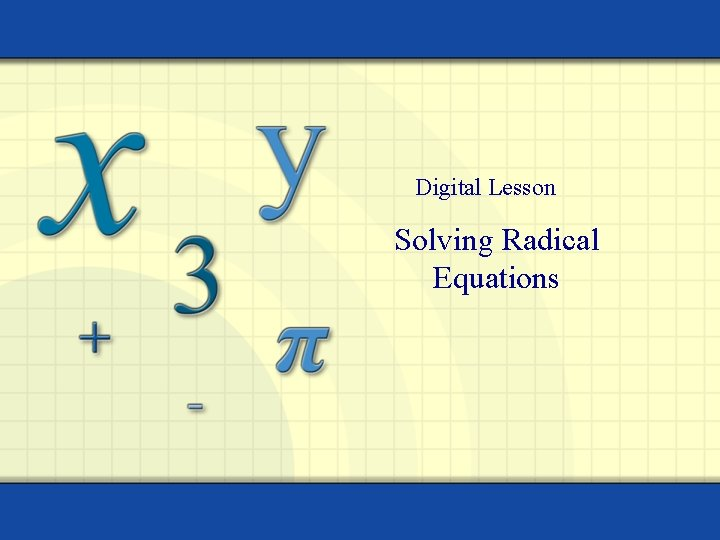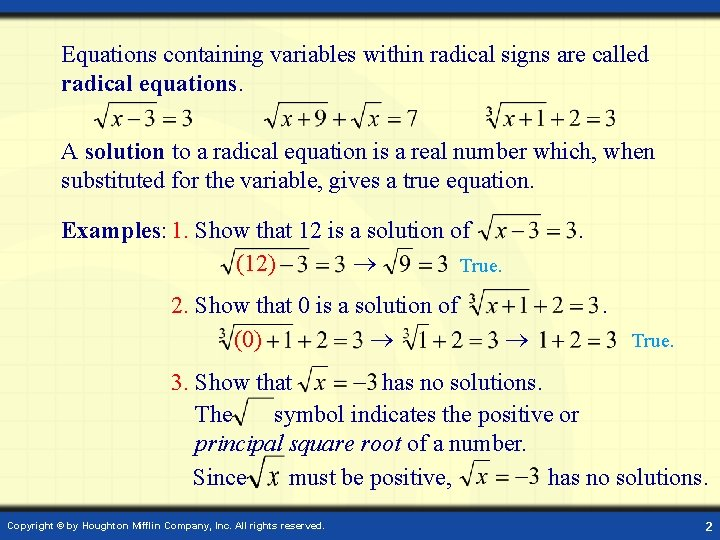Equations containing variables within radical signs are called radical equations. A solution to a radical equation is a real number which, when substituted for the variable, gives a true equation. Examples: 1. Show that 12 is a solution of (12) True. 2. Show that 0 is a solution of (0) . . True. 3. Show that has no solutions. The symbol indicates the positive or principal square root of a number. Since must be positive, has no solutions. Copyright © by Houghton Mifflin Company, Inc. All rights reserved. 2If a and b are real numbers, n is a positive integer, and a = b, then an = bn This principle can be applied to solve radical equations. Examples: 1. If , then x = 4. 2. If , then x = 125. The converse of the statement is true for odd n and false for even n. If n is odd and a n = b n then a = b. If n is even and a n = b n then it is possible that a b. Example: ( 3)2 = 9 = (3)2 but, 3 3. Copyright © by Houghton Mifflin Company, Inc. All rights reserved. 3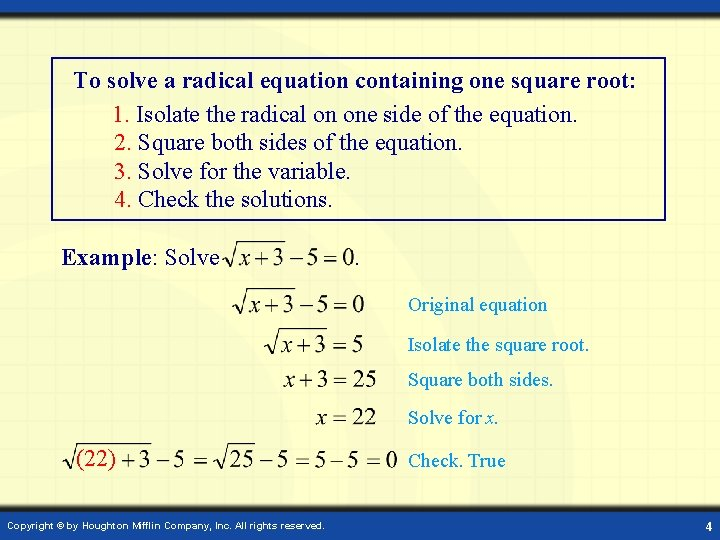To solve a radical equation containing one square root: 1. Isolate the radical on one side of the equation. 2. Square both sides of the equation. 3. Solve for the variable. 4. Check the solutions. Example: Solve . Original equation Isolate the square root. Square both sides. Solve for x. (22) Copyright © by Houghton Mifflin Company, Inc. All rights reserved. Check. True 4Example: Solve . Original equation Isolate the square root. Square both sides. Solve for x. Solutions (1) Check. True (9) Check. True Copyright © by Houghton Mifflin Company, Inc. All rights reserved. 5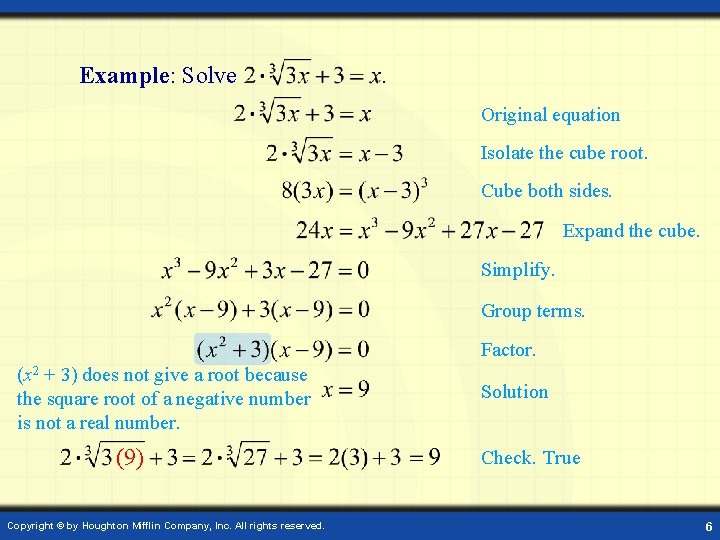Example: Solve . Original equation Isolate the cube root. Cube both sides. Expand the cube. Simplify. Group terms. Factor. (x 2 + 3) does not give a root because the square root of a negative number is not a real number. (9) Copyright © by Houghton Mifflin Company, Inc. All rights reserved. Solution Check. True 6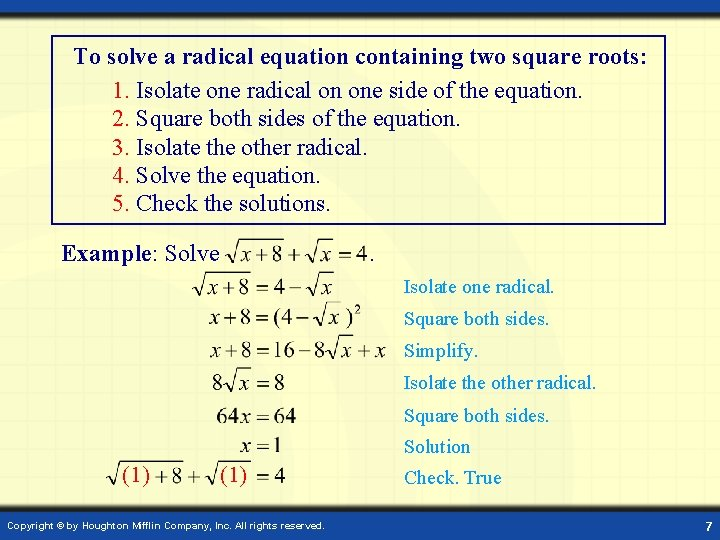To solve a radical equation containing two square roots: 1. Isolate one radical on one side of the equation. 2. Square both sides of the equation. 3. Isolate the other radical. 4. Solve the equation. 5. Check the solutions. Example: Solve . Isolate one radical. Square both sides. Simplify. Isolate the other radical. Square both sides. Solution (1) Copyright © by Houghton Mifflin Company, Inc. All rights reserved. Check. True 7Example: Solve . Original equation Isolate the square root. Square both sides. Simplify. Factor. Possible solutions (2) Check 2. True 2 is a solution of the original radical equation. (14) Check 14. False 14 is not a solution of the original radical equation. Copyright © by Houghton Mifflin Company, Inc. All rights reserved. 8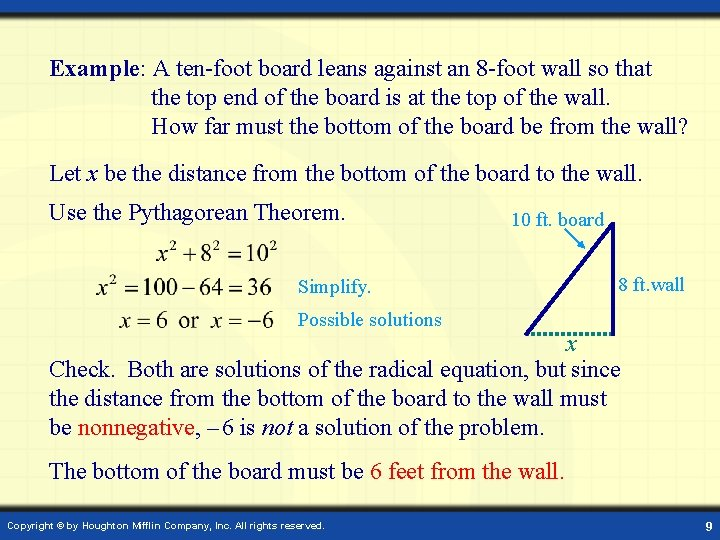Example: A ten-foot board leans against an 8 -foot wall so that the top end of the board is at the top of the wall. How far must the bottom of the board be from the wall? Let x be the distance from the bottom of the board to the wall. Use the Pythagorean Theorem. 10 ft. board Simplify. 8 ft. wall Possible solutions x Check. Both are solutions of the radical equation, but since the distance from the bottom of the board to the wall must be nonnegative, – 6 is not a solution of the problem. The bottom of the board must be 6 feet from the wall. Copyright © by Houghton Mifflin Company, Inc. All rights reserved. 9Example: The time T (in seconds) taken for a pendulum of length L (in feet) to make one full swing, back and forth, is given by the formula To the nearest hundredth, how long is a pendulum which takes 2 seconds to complete one full swing? (3. 24) Radical equation Isolate the radical. Square both sides. Check. True. A pendulum of length approximately 3. 24 feet will make one full swing in 2 seconds. Solve for L. (to the nearest hundredth) Copyright © by Houghton Mifflin Company, Inc. All rights reserved. 10